# R语言中贝叶斯网络（BN）、动态贝叶斯网络、线性模型分析错颌畸形数据

## 贝叶斯网络（BN）是一种基于有向无环图的概率模型，它描述了一组变量及其相互之间的条件依赖性。

1. 预处理数据;
2. 学习贝叶斯网络的结构和参数。
3. 使用网络作为预测模型。
4. 使用网络进行推理。
5. 通过与外部信息的对比来验证网络的有效性。

## 快速介绍

### 贝叶斯网络

• 一个网络结构，一个有向无环图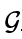, 其中每个节点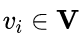对应于一个随机变量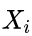;
• 一个全局概率分布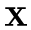(带参数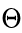), 它可以根据图中存在的弧被分解成更小的局部概率分布。

R实现了以下学习算法。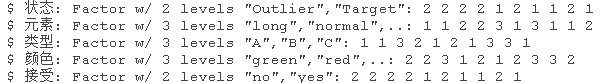``> plot(hc_simd)``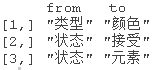``simd_fitted``

# R语言BUGS/JAGS贝叶斯分析: 马尔科夫链蒙特卡洛方法（MCMC）采样

## 错颌畸形数据的贝叶斯网络分析

### 随时关注您喜欢的主题

1. 我们学习一个BN，并使用它来确定和可视化在成长和治疗过程中各种III类错位颌面特征之间的相互作用。
2. 我们通过验证一些普遍接受的关于这些骨骼不平衡演变的假说来检验其一致性。
3. 我们表明，与接受快速上颌扩张和面罩治疗的正畸患者相比，未经治疗的受试者形成了不同的III类颅面生长模式。
4. 在接受治疗的患者中，CoA段（上颌骨长度）和ANB角（上颌骨与下颌骨的前后关系）似乎是接受治疗的主要影响的骨骼亚空间。

### 数据

• `治疗：未经治疗（NT），治疗后效果不好（TB），治疗后效果好（TG）。`
• `生长：一个二元变量，数值为好或坏。`
• `ANB：唐氏点A和B之间的角度（度）。`
• `IMPA：门牙-下颌平面角（度）。`
• `PPPM：腭平面-下颌平面的角度（度）。`
• `CoA：上颌骨从髁状突到唐氏点A的总长度（mm）。`
• `GoPg：下颌体从齿龈到齿龈的长度（mm）。`
• `CoGo：下颌骨的长度，从髁状突到齿状突（mm）。`

`所有的测量都是通过X射线扫描得出的，使用一套参考点建立的图，如以下。`

``> str(data)``

# 生长和治疗变量带有关于病人预后的冗余信息，这一点从TB和TG之间生长良好的病人比例的差异中可以看出。

``````+   hist(x, prob = TRUE )
+   lines(density(x), lwd = 2 )``````

``> pairs(diff\[, setdiff(names(diff)``

``> heatmap(rho)``

# 我们可以在热图中看到两个集群：第一个集群包括dCoGo、dGoPg和dCoA，第二个集群包括Treatment、dANB和dCoA。

``plot(ug )``

# 学习贝叶斯网络

## 学习结构

• 我们把任何指向正畸变量中的dT、治疗和生长的弧列入黑名单。
• 我们将从dT到Treatment的弧列入黑名单。这意味着一个病人是否被治疗不会随时间而改变。
• 我们将从生长到dT和治疗的弧线列入黑名单。这意味着病人是否接受治疗不会随时间变化，而且显然不会因预后而变化。

• 我们将依赖结构dANB → dIMPA ← dPPPM列入白名单。
• 我们将从dT到Growth的弧线列入白名单，这使得预后可以随时间变化。

`至于绘图，关键函数是plot()。`

``plot(dag, , highlight )``

1. 使用bootstrap对数据重新取样。
2. 从每个bootstrap样本中学习一个单独的网络。
3. 检查每个可能的弧在网络中出现的频率。
4. 用出现频率较高的弧构建一个共识网络。
``booth(diff, R = 200)``

boot.strength()的返回值包括，对于每一对节点，连接它们的弧的强度（例如，我们观察到dANB → dPPPM或dPPPM → dANB的频率）及其方向的强度（例如，当我们观察到dANB和dPPPM之间有弧时，我们观察到dANB → dPPPM的频率）。

``attr( "threshold")``

``> avg.diff = averaged.network(str.diff)``

``> strength.plot(avg.diff, str.diff, shape = "ellipse", highlight = list(arcs = wl))``

``````> par(mfrow = c(1, 2))
> graphviz.compare(avg.diff, dag, shape = "ellipse", main = c("averaged DAG", "single DAG"))``````

``> compare``

``````> plot(str.diff)
> abline(v = 0.75, col = "tomato", lty = 2, lwd = 2)
> abline(v = 0.85, col = "steelblue", lty = 2, lwd = 2)``````

``````> nrow( strength >  "threshold" direction > 0.5, \])
\[1\] 18
trength > 0.75 &  direction > 0.5
\[1\] 15
strength > 0.85 &  direction > 0.5
\[1\] 12``````

``````> avg.simpler = averaged.network(str.diff, threshold = 0.85)
> strength.plot(avg.simpler, str.diff, shape = "ellipse", highlight = list(arcs = wl))``````

# 学习参数

``fit(avg, diff)``

`` > summary(lm(dANB ~ Growth + Treatment, data = diff))``

``````> # 逐渐增加解释变量之间的关联性。
> for (rho 5)) {

+   # 更新相关矩阵并生成数据。
+   R  = R = rho
+   data = as.data.frame(mvrnorm(1000))
+   # 比较线性模型
+   cat( " BIC:",

+ }``````

# 比较线性模型

`````` dANB

dANB = penalized( dANB)
dANB``````

# 模型验证

1. 只看网络结构：如果学习BN的主要目标是识别弧和路径，当BN被解释为因果模型时，通常是这种情况，我们可以进行本质上的路径分析和研究弧的强度。
2. 将BN视为一个整体，包括参数：如果学习BN的主要目标是将其作为一个专家模型，那么我们可能想。
• 根据其他一些变量的值，预测新个体的一个或多个变量的值；以及
• 将CP查询的结果与专家知识进行比较，以确认BN反映了关于我们正在建模的现象的最佳知识。

# 预测准确性

• _k-fold交叉验证_;
• 指定的k进行交叉验证;
• hold-out 交叉验证

• 结构学习算法（结构和参数都是从数据中学习的）。
• 参数学习算法（结构由用户提供，参数从数据中学习）。

``````cv(diff)

> for (i in 1:10) {

+   err\[i\] = (sum(tt) - sum(diag(tt))) / sum(tt)

+ }
>``````

``````> for (var in names(predcor)) {

+   xval = cv(diff)

+     predcor\[var\] = mean(sapply(xval, function(x) attr(x, "mean")))

+ }``````

## 用专家知识进行确认

“CoGo的过度增长应该会引起PPPM的减少”。

``> sim = dist(fitted.simpler) > plot(sim ) > abline(v = 0, col = 2, lty = 2, lwd = 2)``

“CoGo的小幅增长应该会引起PPPM的增长。” 从上图来看，CoGo的负增长或空增长（dCoGo ⋜ 0）对应于PPPM的正增长，概率为≈0.60。对于CoGo的小幅增长（dCoGo∈[0, 2]），不幸的是，dPPPM ⋜0，概率≈0.50，所以BN不支持这一假设。

``> nrow(sim\[( dCoGo <= 0) & ( PPPM > 0), \]) / nrow(sim\[( dCoGo <= 0), \]) \[1\] 0.6112532 > nrow(sim\[( dCoGo > 0) & ( dCoGo < 2) & ( dPPPM > 0), \]) / +   nrow(sim\[( CoGo) > 0 & ( dCoGo < 2),  \]) \[1\] 0.4781784``

“如果ANB减少，IMPA就会减少以进行补偿。” 像以前一样通过模拟测试，我们正在寻找与IMPA（相同）的负值相关的dANB的负值（这表明假设角度最初是正的，就会减少）。从下图中可以看出，dANB与dIMPA成正比，所以其中一个的减少表明另一个的减少；两者的平均趋势（黑线）同时为负。

``> plot(sim ) > abline(coef(lm(dIMPA ~ dANB ))``

“如果GoPg强烈增加，那么ANB和IMPA都会减少。” 如果我们从BN中模拟dGoPg、dANB和dIMPA，假设dGoPg>5（即GoPg在增加），我们估计dANB>0（即ANB在增加）的概率为≈0.70，dIMPA<0的概率仅为≈0.58。

``> nrow(sim\[(dGoPg > 5) & (dANB < 0), \]) / nrow(sim\[(dGoPg > 5), \]) \[1\] 0.695416 > nrow(sim\[(dGoPg > 5) & (dIMPA < 0), \]) / nrow(sim\[(dGoPg > 5), \]) \[1\] 0.5756936``

_”治疗试图阻止ANB的减少。如果我们固定ANB，治疗过的病人和未治疗过的病人是否有区别？”`首先，我们可以检查在没有任何干预的情况下，dANB≈0的病人的治疗和增长之间的关系（即使用我们从数据中得知的BN）。`

``_ dist(fitted&nbsp;) table(TREATMENT&nbsp;=&nbsp;Treatment&nbsp;&lt;&nbsp;0.5,&nbsp;GOOD.GROWTH&nbsp;=&nbsp;&nbsp;Growth&nbsp;&gt;&nbsp;0.5)``

`` table(TREATMENT&nbsp;=&nbsp;&nbsp;Treatment&nbsp;&lt;&nbsp;0.5,&nbsp;GOOD.GROWTH&nbsp;=&nbsp;&nbsp;Growth&nbsp;&gt;&nbsp;0.5)``
1. _”治疗试图阻止ANB的减少。如果我们固定ANB，治疗和未治疗的病人之间是否有区别？”`评估的方法之一是检查在保持GoPg固定的情况下，A点和B点之间的角度（ANB）是否在治疗和未治疗的病人之间发生变化。_`

``````Treatment = c("UNTREATED", "TREATED")\[(Treatment > 0.5) + 1L\]
boxplot(dANB ~ Treatment)``````

# 模型#2：动态贝叶斯网络

## 学习结构

``> t2.variables``

1. 我们将所有从临床变量到T1、T2和治疗的弧线列入黑名单，因为我们知道，年龄和治疗不是由临床测量决定的。
2. 我们将所有进入Treatment和t1时间段的所有变量的弧列入黑名单，因为我们假设t1时间段的变量之间的弧与t2时间段的相应变量是一样的，两次学习它们是没有意义的。
3. 我们将所有从t2到t1的弧列入黑名单。
``````grid(from = setdiff(names(ortho), c("T1", "T2")),
to = c("T1", "T2"))``````

``>  data.frame(from = c("T1"), to = c("T2"))``

``> dyn.dag``

``plot(dyn, render = FALSE)``

``````+        attrs = list(graph = list(rankdir = "LR")))

> Graph(gR)``````

# 结构学习中的模型平均化

``````> boot (ortho )
> plot(dyn)``````
``avernet(dyn.str)``

``````compare(dag, avg)
tp fp fn
26  1  1``````

# 学习参数

``fit(dynavg)``

### 模型验证和推理

“在不同的治疗下，ANB从第一次测量到第二次测量的转变程度如何？”

``data.frame(  diff = c(nt\[, 2\] - nt\[, 1\], tb\[, 2\] - tb\[, 1\], tg\[, 2\] - tg\[, 1\]), > by(effect\$diff, effect\$treatment, FUN = mean)``

``````> for (i in seq(nrow(interv)) {
+   # 进行联合预测，目前用predict()无法实现。
+   dist(dyn.fitted, nodes = c(),
+   intervals\[i,\] = weighted.mean(ANB2, weights)
+   intervals\[i,\] = weighted.mean(CoA2, weights)``````

Kaizong Ye拓端研究室（TRL）的研究员。

​非常感谢您阅读本文，如需帮助请联系我们！QQ在线咨询

15121130882

0571-63341498

## 关注有关新文章的微信公众号

This will close in 0 seconds